##CS 660: Combinatorial Algorithms Review of Mathematical Analysis of Algorithms

[To Lecture Notes Index]
San Diego State University -- This page last updated 8/29/95## References

Introduction To Algorithms, Corman, Leiserson,Rivest, Chapters 1-4

## Mathematical Analysis of Algorithms

### Model of Computing

If analysis of algorithms is the answer, what is the question?

Given two or more algorithms for the same task, which is better?
Under which condition is bubble sort better than insertion sort?

What computing resources does an algorithm require?
How long will it take bubble sort to sort a list of N items?

Goal of mathematical analysis is a function of the resources required of an algorithm

On what computer?
What is a Computer?
Random-access machine (RAM)
Single processor
Instructions executed sequentially
Each operation requires the same amount of time

Single cost vs. Lg(N) cost
Time required for basic operation?
3 + 6
1234!
Insertion Sort
A = - infinity
for K = 2 to N do
begin
J = K;
Key = A[J];
while Key < A[J-1] do
begin
A[J] = A[J-1];
J = J - 1;
end while;
A[J] = Key;
end for;
Complexity
Resources required by the algorithm as a function of the input size

Worst-case Analysis
Complexity of an algorithm based on worst input of each size

Average-case Analysis
Complexity of an algorithm averaged over all inputs of each size
Insertion Sort
 Comparisons Element moves worst case (N+1)N/2 - 1 (N-1)N/2 average case (N+1)N/4 - 1/2 (N-1)N/4

### Asymptotic Notation

Asymptotically tight bound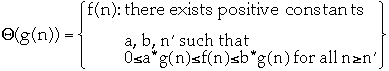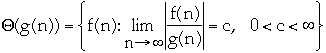Asymptotic upper bounds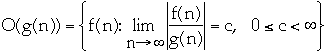Common Myths and Errors
• Everyone incorrectly writes: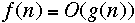instead of: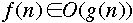•ordoes not mean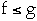or even that there is an n such that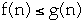Let f(n) = 2n + 10, and g(n) = n then
f(n) = O(g(n)) but f(n) > g(n)

• Using O( ) when meanBubble vs. Insertion Sort
 Worst case Average case Bubble sort Insertion Sort

Bubble Sort
 Comparisons Element moves worst case (N-1)N/2 3(N-1)N/2 average case (N-1)N/2 3(N-1)N/4 best case (N-1)N/2 0

Insertion Sort
 Comparisons Element moves worst case (N+1)N/2 - 1 (N-1)N/2 average case (N+1)N/4 - 1/2 (N-1)N/4 best case N - 1 0
Bubble vs. Insertion SortTiming Results
Worst Case
 N Bubble Insertion 100 1 1 200 5 3 400 19 11 800 79 42 1600 317 166

Average Case
 N Bubble Insertion 100 1 0 200 3 1 400 14 5 800 56 21 1600 228 84

What is wrong with this Picture?

## Timing Analysis

### Timing in C on Rohan

main()
{
int k, iterations;
for (iterations = 0; iterations < 50; iterations++)
{
start();
/* start the timer */
for (k = 0; k < 2000000; k++)
/* do some work */
k = k;
stop();
/* stop the timer */
printf("Time taken: %ld\n", report());
};
}
Result on Rohan

Time Frequency Occurred
30 2
31 2
32 9
33 10
34 11
35 9
36 5
37 1
39 1
Source for Timing C Code on Rohan

#include <stdio.h>
#include <sys/times.h>
#include <limits.h>

static struct tms _start; /* Stores the starting time*/
static struct tms _stop; /* Stores the ending time*/

int start()
{
times(&_start);
}

int stop()
{
times(&_stop);
}

unsigned long report()
{
return _stop.tms_utime - _start.tms_utime;
}

main()
{
int k, iterations;
for (iterations = 0; iterations < 50; iterations++)
{
start();
/* start the timer */
for (k = 0; k < 2000000; k++)
/* do some work */
k = k;
stop();
/* stop the timer */
printf("Time taken: %ld\n", report());
};
}

### Handling Measurement Errors

Repeat a measurement n times
Let the measurements be labeled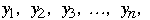Letand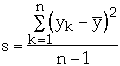The confidence interval for the true measurement is:The value of t determine the probability the measurement is in the interval

When n >= 50
Probability value of t
 50% 80% 90% 95% 99% 0.67 1.28 1.64 1.96 2.58
In Example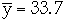, s = 3.15, selecting t = 1.96 we get95% confidence interval is (32.83, 34.57)
Student t table - When n < 50
 n 90% 95% 99% 1 3.078 6.314 31.821 2 1.886 2.920 6.965 3 1.638 2.353 4.541 4 1.533 2.132 3.747 5 1.476 2.015 3.365 6 1.440 1.943 3.143 7 1.415 1.895 2.998 8 1.397 1.860 2.896 9 1.383 1.833 2.821 10 1.372 1.812 2.764 20 1.325 1.725 2.528 30 1.310 1.697 2.457 40 1.303 1.684 2.423

### Estimating Complexity from Timing Results

Fun with Functions

Let f(n) = 3n*n + 4n + 5 and g(n) = 3n*n

Fact: g(n) is an approximation of f(n)

Notation: f(n) = g(n) +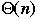n f(n) g(n) % error 1 12 3 75.00% 10 345 300 13.04% 20 1285 1200 6.61% 30 2825 2700 4.42% 40 4965 4800 3.32% 50 7705 7500 2.66% 60 11045 10800 2.22% 70 14985 14700 1.90% 80 19525 19200 1.66% 90 24665 24300 1.48% 100 30405 30000 1.33% 200 120805 120000 0.67% 300 271205 270000 0.44%
Eyeballing Complexity

Letthen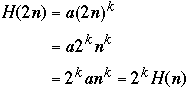Timing Results
 N Bubble Insertion 100 1 1 200 5 3 400 19 11 800 79 42 1600 317 166
Plotting Complexity
Cubic or Quadratic?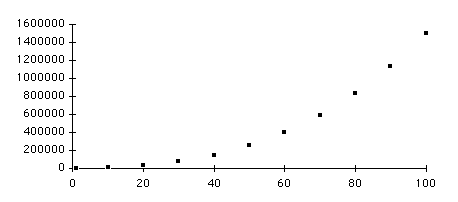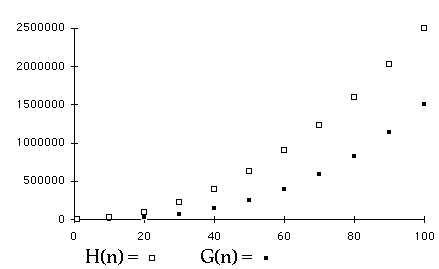Plotting ComplexityEngineers Method (Modified)

Let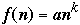then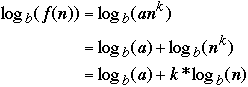Let b = 2 and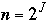thenPlotting ComplexityTransform the Axis

Letand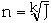(or) then:

g(J) = f(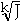) = a()k = aJ

So g(J) is linear!
Example
 n f(n) =5n*n+n + 3 J=n*n 1 9 1 10 513 100 20 2023 400 30 4533 900 40 8043 1600 50 12553 2500 60 18063 3600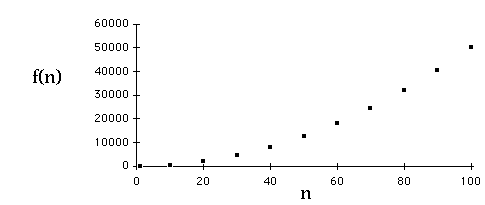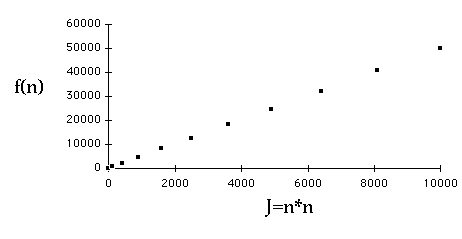Which is Quadratic?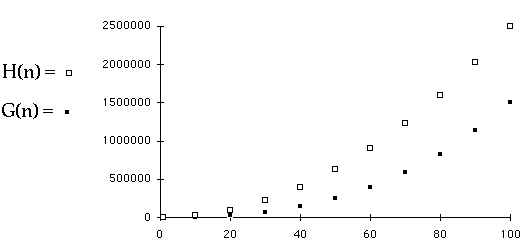### Mathematical Analysis and Timing Code

Bubble sort worst case is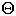( n*n )

Complexity is an*n + bn + c

Timing Results Worst Case
 N Bubble Sort 400 20 500 31 600 45 700 61 800 79

Least Squares fit of data to an*n + bn + c

Bubble sort worst case is 0.0001143n*n + 0.01084n - 2.738

Predicted vs. Actual Time for Bubble Sort
 N Actual Predicted % Error 900 105 99.601 5.14% 1000 124 122.402 1.29% 1100 149 147.489 1.01% 2000 496 476.142 4.00% 2400 713 681.646 4.40%